2022: SklogWiki celebrates 15 years on-line

# Gibbs ensemble

Here we have the N-particle distribution function (Ref. 1 Eq. 2.2)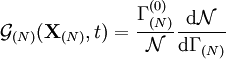$\mathcal{G}_{(N)} ({\mathbf X}_{(N)},t)= \frac{\Gamma_{(N)}^{(0)}}{\mathcal{N}} \frac{{\rm d}\mathcal{N}}{{\rm d}\Gamma_{(N)}}$

where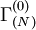$\Gamma_{(N)}^{(0)}$ is a normalized constant with the dimensions of the phase space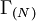$\left. \Gamma_{(N)} \right.$.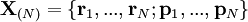${\mathbf X}_{(N)} = \{ {\mathbf r}_1 , ..., {\mathbf r}_N ; {\mathbf p}_1 , ..., {\mathbf p}_N \}$

Normalization condition (Ref. 1 Eq. 2.3):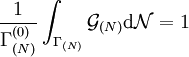$\frac{1}{\Gamma_{(N)}^{(0)}} \int_{\Gamma_{(N)}} \mathcal{G}_{(N)} {\rm d}\mathcal{N} =1$

it is convenient to set (Ref. 1 Eq. 2.4)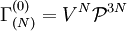$\Gamma_{(N)}^{(0)} = V^N \mathcal{P}^{3N}$

where$V$ is the volume of the system and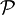$\mathcal{P}$ is the characteristic momentum of the particles (Ref. 1 Eq. 3.26),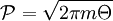$\mathcal{P} = \sqrt{2 \pi m \Theta}$

Macroscopic mean values are given by (Ref. 1 Eq. 2.5)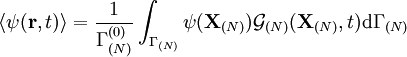$\langle \psi ({\mathbf r},t)\rangle= \frac{1}{\Gamma_{(N)}^{(0)}} \int_{\Gamma_{(N)}} \psi ({\mathbf X}_{(N)}) \mathcal{G}_{(N)} ({\mathbf X}_{(N)},t) {\rm d}\Gamma_{(N)}$

### Ergodic theory

Ref. 1 Eq. 2.6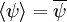$\langle \psi \rangle = \overline \psi$

### Entropy

Ref. 1 Eq. 2.70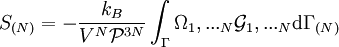$S_{(N)}= - \frac{k_B}{ V^N \mathcal{P}^{3N}} \int_\Gamma \Omega_1,... _N \mathcal{G}_1,... _N {\rm d}\Gamma_{(N)}$

where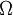$\Omega$ is the N-particle thermal potential (Ref. 1 Eq. 2.12)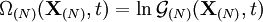$\Omega_{(N)} ({\mathbf X}_{(N)},t)= \ln \mathcal{G}_{(N)} ({\mathbf X}_{(N)},t)$